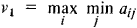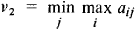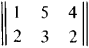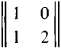# Matrix Games

Also found in: Wikipedia.

## Matrix Games

a concept in game theory. Matrix games are games played by two players (I and II) with opposing interests, each player having a finite number of pure strategies. If player I has m strategies and player II has n strategies, then the game may be defined by the (m × n) matrix A = ║aij║, where aij is the payoff paid to player I by player II if player I selects strategy i (i = 1, …, m) and player II selects strategy j (j = 1, …, n). Player I, following the general principles of behavior in zero-sum games (of which the matrix game is a particular case) strives to select a strategy i0 for which the maximum is achieved inand player II strives to select a strategy j0 for which the minimum is achieved inIf v1 = v2, then the pair (i0, j0) constitutes a saddlepoint of the game, that is, the double inequality

aij0a10j0ai0j, i = 1, …, m, j = 1, …, n

is fulfilled. The number ai0j0 is called the value of the game, and the strategies i0 and j0 are called the optimal pure strategies of players I and II, respectively. If v1V2, then v1 < v2 always. In this case, no saddlepoint exists in the game, and the optimal strategies of the players must be found from among their mixed strategies (that is, probability distributions on the set of pure strategies). In this case, the players’ choice of strategies are determined by the mathematical expectations of the payoffs.

The principal theorem of the theory of matrix games—the minimax theorem of von Neumann—asserts that in any matrix game there exist optimal mixed strategies x*, y* for which the achieved “minimaxes” are equal (their common value is the value of the game). For example, a game with matrixhas a saddlepoint with i0 = 2, j0 = 1, and the value of the game is equal to 2. A game with matrixhas no saddlepoint; the optimal mixed strategies for it are x* = (¾, ¼), y* = (½, ½), and the value of the game is ½.

The possibility of reducing a matrix game to linear programming problems is most often used to actually find the optimal mixed strategies. It is possible to use what is called the Brown-Robinson iteration method, which consists of successive fictitious “playing out” of the given game during which each player in turn selects his own pure strategies that would have been optimal against the strategies selected by the opponent up to this time. Games in which one of the players has only two strategies are solved simply by graphs.

Matrix games can be used as mathematical models of many simple situations of conflict in economics, mathematical statistics, military science, and biology. Often “nature” is considered as one of the players, where by “nature” is understood the entire set of external facts unknown to the individual undertaking a solution (who is considered as the other player).

### REFERENCES

Matrichnye igry. Edited by N. N. Vorob’ev. Moscow, 1961. (Collection of translated articles.)
Von Neumann, J., and O. Morgenstern. Teoriia igr i ekonomicheskoe povedenie. Moscow, 1970. (Translated from English.)
Owen, G. Teoriia igr. Moscow, 1971. (Translated from English.)

A. A. KORBUT

References in periodicals archive ?
For the purposes of synthesis of real control algorithms, simplified game models in the form of positional and matrix games are used.
Sakawa and Nishizaki , Kumar [ 12]studied single and multi objective matrix games with fuzzy goals and fuzzy payoff by using max-min principle of game theory.
The authors cover combination games, normal play games, impartial games, Hackenbush and Partizan games, Von NeumannAEs minimax theorem, zero-sum matrix games, n-player games, cooperation, and a great many other related subjects.
In many cases, their computation is tractable (e.g., Papadimitriou (2005); Papadimitriou and Roughgarden (2005)), whereas computing Nash equilibria is PPAD-complete even for 2-player matrix games (see Chen and Deng (2005); Daskalakis et al.
Towards Solving Matrix Games with Interval Type-2 Fuzzy Uncertainty through Linear Optimization
(2005) Fuzzy matrix games multi-criteria model for decisionmaking in engineering, Informatica, 16(1), pp.
Their topics include financial markets, polynilpotent multipliers of finitely generated abelian groups, the concept of subtype in Bernstein algebras, optimal processes in irreversible microeconomics, algebraic solutions for matrix games, methods of drawing special curves and surfaces, some game theory and financial contracting issues in large corporate transactions, endomorphisms and endomorphism semigroups of groups, the noiseless coding theorem, odd zeta and other special function bounds, and spectral properties of discrete Schrodinger operator with quasi-periodically recurrent potential.
In view of (7) like of the case of scalar matrix games the following theorem is proving.
1994); matrix games in construction technology and management (Peldschus and Zavadskas 1997); game theory in construction technology and management (Zavadskas et al.
ERIC YOUNG'S SQUAD ASSAULT: WESTFRONT, Matrix Games, PC, pounds 19.99
Gonzalez, Fuzzy matrix games considering the criteria of players, Kybernetes 20 (1991), 145-153.
So, four distinct matrix games are to be considered: [[Gamma].sub.ll], [[Gamma].sub.lh], [[Gamma].sub.hl], and [[Gamma].sub.hh].

Site: Follow: Share:
Open / Close